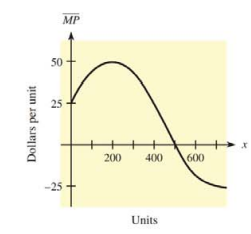Chapter 10, Problem 37RE### Mathematical Applications for the ...

11th Edition
Ronald J. Harshbarger + 1 other
ISBN: 9781305108042

#### Solutions

Chapter
Section### Mathematical Applications for the ...

11th Edition
Ronald J. Harshbarger + 1 other
ISBN: 9781305108042
Textbook Problem

# Marginal profit The figure shows the graph of a marginal profit function for a company. At what level of sales will profit be maximized? Explain.To determine

The level of sales where the profit is maximum and the figure shows the graph of a marginal profit function for a company,Explanation

Given Information:

The provided graph is

Explanation:

Consider the graph

The figure represents the graph of a marginal profit function for a company.

Recall that the profit is maximized when the marginal profit curve changes from positive to negative.

From the graph, the curve is increasing before x=200 and after x=200 the curve is decreasing but at

### Still sussing out bartleby?

Check out a sample textbook solution.

See a sample solution

#### The Solution to Your Study Problems

Bartleby provides explanations to thousands of textbook problems written by our experts, many with advanced degrees!

Get Started